《我的世界》1.8版智能化僵尸四个技能介绍及对抗技巧攻略

《我的世界》1.8版游戏中命令方块智能化僵尸四个技能分别为什么？该怎么对抗？下面给大家带来僵尸四个技能介绍及对抗技巧攻略。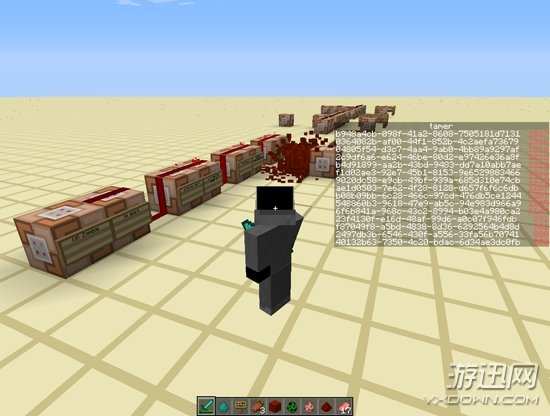replace entity @e[type=Zombie] slot.armor.head minecraft:skull 1 2

execute @a ~ ~ ~ scoreboard players add @e[type=Zombie,r=20] tamer 1

execute @e[type=Zombie,score_tamer_min=900] ~ ~ ~ summon Giant ~ ~ ~

tp @e[score_tamer_min=900,type=Zombie] ~ ~-600 ~

execute @a ~ ~ ~ execute @e[r=5,type=Zombie] ~ ~ ~ tp @e[type=Zombie,r=20] @r[r=5]

execute @a ~ ~ ~ execute @e[r=5,type=Giant] ~ ~ ~ tp @e[type=Giant,r=20] @a[r=5]

execute @a ~ ~ ~ execute @e[r=5,type=Giant] ~ ~ ~ tp @e[type=Zombie,r=20] @a[r=5]

execute @a ~ ~ ~ execute @e[r=5,type=Zombie] ~ ~ ~ tp @e[type=Giant,r=20] @a[r=5]

execute @e[type=Zombie] ~ ~ ~ kill @e[type=Pig,r=5]

execute @e[type=Pig] ~ ~ ~ effect @e[type=Zombie,r=5] 7 1 1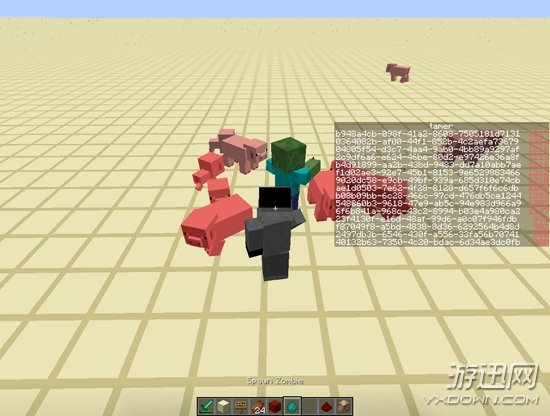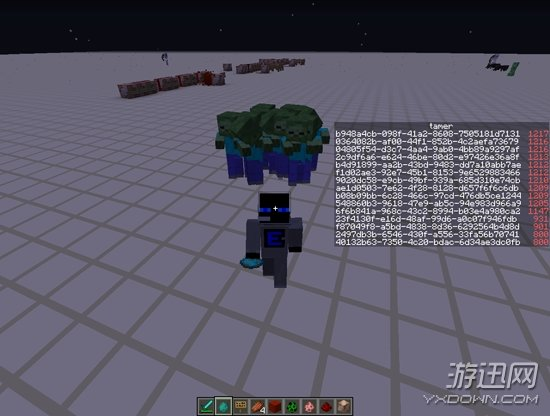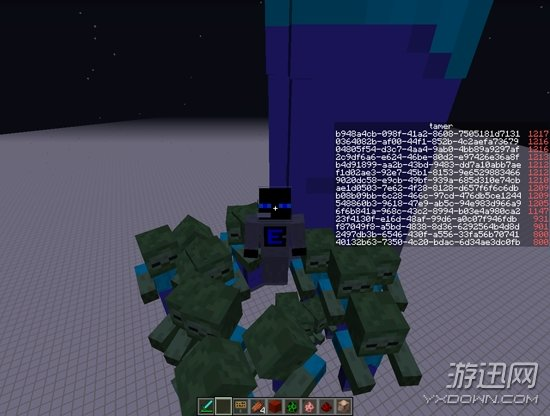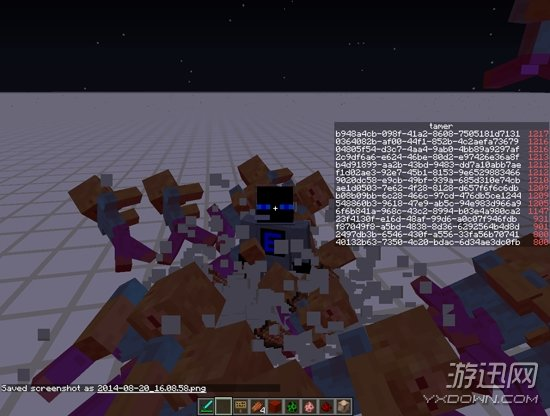1. 下载悟饭手机客户端
2. 安装悟饭手机客户端到手机中
3. 在手机客户端中找到游戏并下载
4. 下载游戏完成后开始游戏